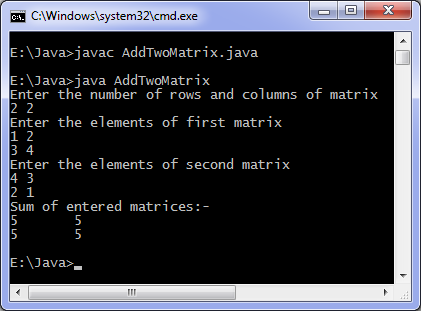# Java program to add two matrices

Levels of difficulty: / perform operation:

This java program add two matrices. You can add matrices of any order.

## This java program

```import java.util.Scanner;
public static void main(String args[]) {
int m, n, c, d;
Scanner in = new Scanner(System.in);
System.out.println("Enter the number of rows and columns of matrix");
m = in.nextInt();
n  = in.nextInt();
int first[][] = new int[m][n];
int second[][] = new int[m][n];
int sum[][] = new int[m][n];
System.out.println("Enter the elements of first matrix");
for (  c = 0 ; c < m ; c++ )
for ( d = 0 ; d < n ; d++ )
first[c][d] = in.nextInt();
System.out.println("Enter the elements of second matrix");
for ( c = 0 ; c < m ; c++ )
for ( d = 0 ; d < n ; d++ )
second[c][d] = in.nextInt();
for ( c = 0 ; c < m ; c++ )
for ( d = 0 ; d < n ; d++ )
sum[c][d] = first[c][d] + second[c][d];
//replace '+' with '-' to subtract matrices
System.out.println("Sum of entered matrices:-");
for ( c = 0 ; c < m ; c++ ) {
for ( d = 0 ; d < n ; d++ )
System.out.print(sum[c][d]+"\t");
System.out.println();
}
}
}
```

### OutputThis code adds two matrix, you can modify it to add any number of matrices. You can create a Matrix class and create it’s objects and then create an add method which sum the objects, then you can add any number of matrices by repeatedly calling the method using a loop.